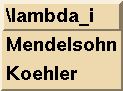´

The parameters of a design have to fulfil certain restrictions. They arise from the fact that a t-design D is also a s-design for any s <= t.

In order to proof this, take an arbitrary s-subset S in the point set V and count the number of pairs (T, B) where T is a t-subset of V containing S, B is a block of the t-design containing T. We have two possibilities to count:

• At first, there are (v-s \choose t-s) possible ways to choose T as a superset of S. For any such T we have lambda blocks B in the design containing T.
• On the other hand, the number of blocks B containing S is equal to the index lambdas and for each such block we can find (k-s \choose t-s) t-subsets T between S and B.
Thus we have the following (equivalent) equations:

lambda · (v-s \choose t-s) = lambdas · (k-s \choose t-s)

lambdas = lambda · (v-s \choose t-s) / (k-s \choose t-s)

So D is an s-(v, k, lambdas) design for all s <= t.

But this equation (actually we have t+1 equations - one for each s in {0, ..., t}) yields restrictions on the parameter sets {t, v, k, lambda}, because all the lambdas have to be integers. A parameter set which fulfils these restrictions is called admissible parameter set.

Let delta_lambda be the lcm of the denominators in the second equation above for all s <= t. Then all parameter sets of the form t-(v, k, h · delta_lambda) with any natural number h are admissible. Thus the calculation of delta_lambda is essential. In order to do that with DISCRETA, you have to press the button "Parameters" and receive the following table:By choosing "\lambda_i" you start the calculation of all the lambdas and delta_lambda. They are shown in the DISCRETA-window as well as on the terminal, where you started DISCRETA. Two short examples:

• the parameters 2-(15, 3, 1) yield the output

v=15 t=2 k=3 lambda=1
t'=1 lambda'=7/1 delta_lambda=1
t'=0 lambda'=35/1 delta_lambda=1

The lambda' are all integral and delta_lambda=1, so this parameter set is admissible and even the parameter sets 2-(15, 3, lambda) with a natural number lambda is admissible.

• the parameters 2-(15, 7, 1) yield the output

v=15 t=2 k=7 lambda=1
t'=1 lambda'=7/3 delta_lambda=3
t'=0 lambda'=5/1 delta_lambda=3

So the parameters are not admissible, because 7/3 is not integral. But the parameter sets 2-(15, 7, h · 3) are admissible for any natural number h.

Remark: You have to click on the pictures to get them largely.

Back to the description .
Back to the DISCRETA homepage .
Last updated: August 24, 1999, Evi Haberberger

University of Bayreuth -